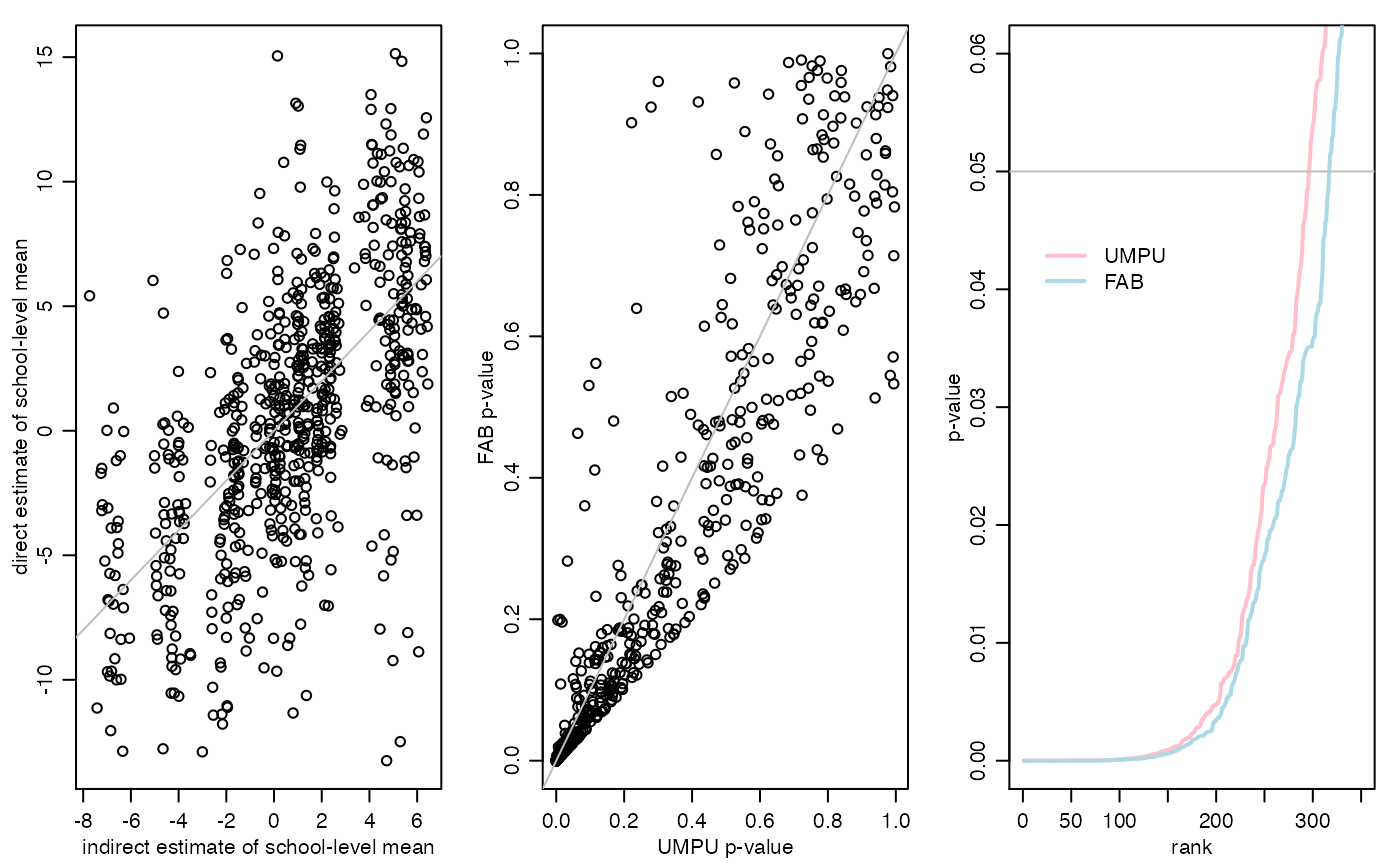## Summary

Adaptive FAB $$p$$-values are constructed for evaluating the mean test scores of 10th graders in a population of high schools. Information is shared across schools using a Fay-Herriot random effects model. This document serves as the replication code for the example in Section 3.2 of the article “Smaller $$p$$-values via indirect information” (Hoff 2019).

## Data

The data for this example are from the 2002 Educational Longitudinal Survey, data from which can be obained at https://nces.ed.gov/surveys/els2002/. A subset of the data needed to replicate the example is available on my website and can be loaded using the following command:

load(url("http://www2.stat.duke.edu/~pdh10/Datasets/els.RData")) 

Now take a look at all the variables:

els[1:10,]
##    school  flp urbanicity sctrl enrollment region female   ses nateng pared
## 1    1011 40.5          1     1          6      1      1 -0.23      1     5
## 2    1011 40.5          1     1          6      1      1  0.69      0     5
## 3    1011 40.5          1     1          6      1      1 -0.68      1     2
## 4    1011 40.5          1     1          6      1      1 -0.89      1     2
## 5    1011 40.5          1     1          6      1      1 -1.28      0     1
## 6    1011 40.5          1     1          6      1      0 -0.93      0     2
## 7    1011 40.5          1     1          6      1      0  0.36      1     6
## 8    1011 40.5          1     1          6      1      0 -0.24      1     2
## 9    1011 40.5          1     1          6      1      0 -0.85      1     2
## 10   1011 40.5          1     1          6      1      0 -1.07      1     1
##    mscore rscore cscore rural suburban urban public catholic private rn rm rs
## 1   52.11  59.53  56.21     0        0     1      1        0       0  1  0  0
## 2   57.65  56.70  57.66     0        0     1      1        0       0  1  0  0
## 3   66.44  64.46  66.50     0        0     1      1        0       0  1  0  0
## 4   44.68  48.69  46.46     0        0     1      1        0       0  1  0  0
## 5   40.57  33.53  36.17     0        0     1      1        0       0  1  0  0
## 6   35.04  28.85  30.72     0        0     1      1        0       0  1  0  0
## 7   50.71  40.80  45.46     0        0     1      1        0       0  1  0  0
## 8   66.17  68.28  68.39     0        0     1      1        0       0  1  0  0
## 9   39.43  45.73  42.07     0        0     1      1        0       0  1  0  0
## 10  46.17  41.05  43.17     0        0     1      1        0       0  1  0  0
## 1   0      0
## 2   0      0
## 3   0      0
## 4   0      0
## 5   0      0
## 6   0      0
## 7   0      1
## 8   0      0
## 9   0      0
## 10  0      0

Separate out student-level characteristics from school-level characteristics:

y<-els$rscore group<-els$school
groups<-sort(unique(group))

W<-as.matrix(els[,c("flp","urban","rural","private","catholic","rm","rs","rw","enrollment") ] )
X<-apply(W,2,function(x){ tapply(x,group,"mean") } ) 

Here, y is the student-level reading score, and group is the school id for each student. That is, group[i] is the school of student with score y[i]. The matrix X is a matrix of school-level variables, and the matrix W is the same data except with the variable for each school replicated for each student in the school.

## UMPU $$p$$-values

Let $$\theta_j$$ be the “true” mean test score in school $$j$$. The standard two-sided $$p$$-value for testing $$H_j:\theta_j=50$$ is based on the $$t$$-statistic $$t_j = \sqrt{n_j}( \bar y_j - 50)/\hat\sigma_j$$.

theta0<-50
YBAR<-tapply(y,group,mean) - theta0
S2<-tapply(y,group,var)
N<-c(table(group))
T<-YBAR/sqrt(S2/N)
pU<-c(2*pt(-abs(T),N-1))

To compute the FAB $$p$$-values, we need to first obtain indirect information about each $$\theta_j$$ using data from schools other than school $$j$$. We quantify this indirect information using the following linking model for school-specific means: $\theta_j = \beta^\top x_j + \tau \gamma_j$ where $$\beta$$ and $$\tau$$ are unknown, $$x_j$$ is a vector of observed characteristics of school $$j$$, and $$\gamma_1,\ldots, \gamma_p \sim$$ are i.i.d. standard normal random variables. For each school $$j$$, we get an estimate of these parameters using the data from schools other than $$j$$, along with an estimate of the within school sampling variance $$\sigma^2$$. This is done using the lmer command in the lme4 package:

library(lme4)
BETA<-TAU<-SIGMA<-NULL
for(i in 1:length(groups)){

g<-groups[i]
iy<-y[group!=g]
iW<-W[group!=g,]
igroup<-group[group!=g]

ifit<-lmer(iy ~ iW + (1|igroup) )

beta<-fixef(ifit)
tau<-sqrt(unlist(VarCorr(ifit)))
sigma<-attr(VarCorr(ifit), "sc")

BETA<-rbind(BETA,beta)
TAU<-c(TAU,tau)
SIGMA<-c(SIGMA,sigma)

#cat(g,"\n")
}

Note that the $$j$$th elements of BETA, TAU and SIGMA are estimated WITHOUT data from school $$j$$, and so are independent of the data in school $$j$$.

## FAB $$p$$-values

Based on these linking model parameter estimates we compute an indirect/prior mean $$\theta_j$$: Given school-level covariates $$x_j$$, the fitted predicted value for $$\theta_j$$ based on the linking model is $$\hat\beta^\top x_j$$:

ETHETA<-apply( BETA*cbind(1,X) ,1,sum) - theta0

Here we have centered things around the hypothesized mean value of $$\theta_0=50$$.

The FAB $$p$$-value for school $$j$$ is $$1-|F( T_j + \tilde b_j ) - F(-T_j)|$$, where $$T_j$$ is the $$t$$ statistic for school $$j$$, $$b_j = 2 \tilde \mu (\tilde \sigma/\sqrt{n_j})/\tilde\tau^2$$, and $$F$$ is the CDF of the appropriate $$T$$-distribution.

pF<- c(1-abs( pt(T+2*ETHETA*(SIGMA/sqrt(N))/(TAU^2),N-1)  - pt(-T,N-1) ) )

Save some results:

resultsFHmodel<-list(T=T,YBAR=YBAR,S2=S2,N=N,pU=pU,ETHETA=ETHETA,TAU=TAU,SIGMA=SIGMA,pF=pF)
save(resultsFHmodel,file="resultsFHmodel.RData")

## Summary of results

sum(pF<=.05)
##  316
sum(pU<=.05)
##  295
mean(pF<=pU)
##  0.7733918
par(mfrow=c(1,3),mar=c(3,3,1,1),mgp=c(1.75,.75,0))

plot(resultsFHmodel$ETHETA,resultsFHmodel$YBAR,
xlab="indirect estimate of school-level mean",
ylab="direct estimate of school-level mean")
abline(0,1,col="gray")

pU<-resultsFHmodel$pU pF<-resultsFHmodel$pF

plot(pU,pF,xlab="UMPU p-value",ylab="FAB p-value")
abline(0,1,col="gray")

plot(sort(pU),type="l",xlim=c(0,350),ylim=c(0,.06),lwd=2,col="pink",
xlab="rank",ylab="p-value")
lines(sort(pF),col="lightblue",lwd=2)
abline(h=.05,col="gray")
legend(5,.045,lwd=c(2,2),col=c("pink","lightblue"),legend=c("UMPU","FAB"),
bty="n")